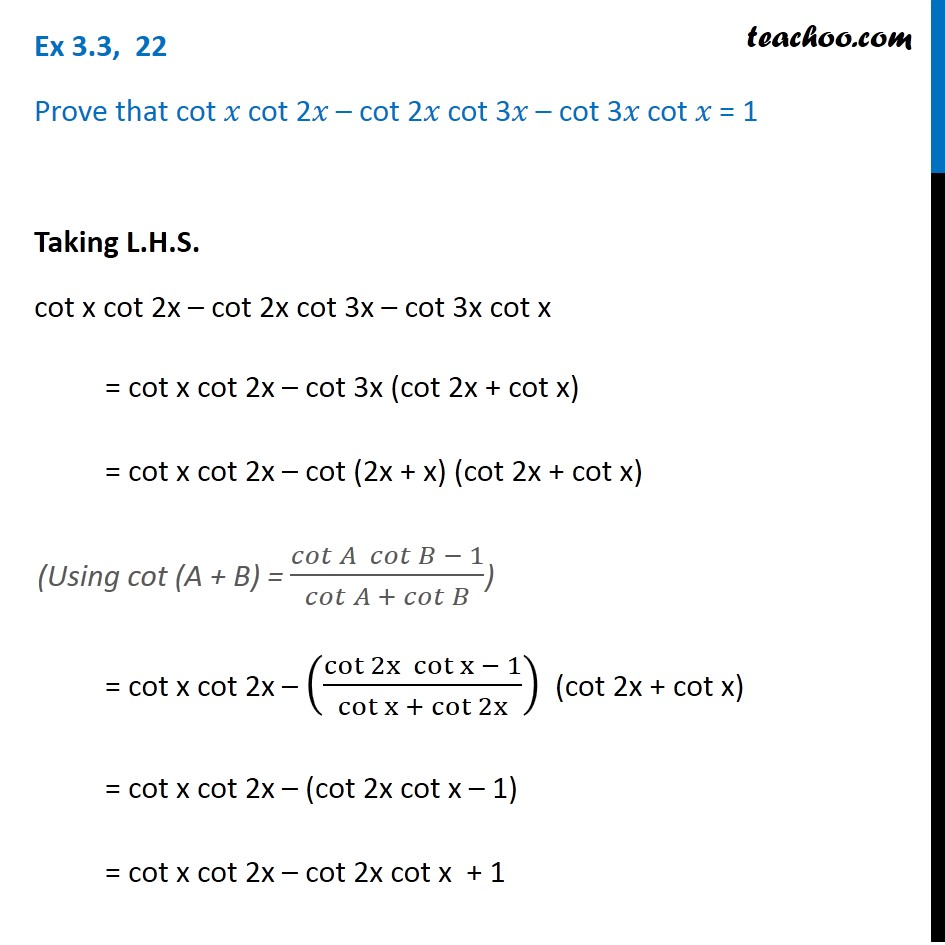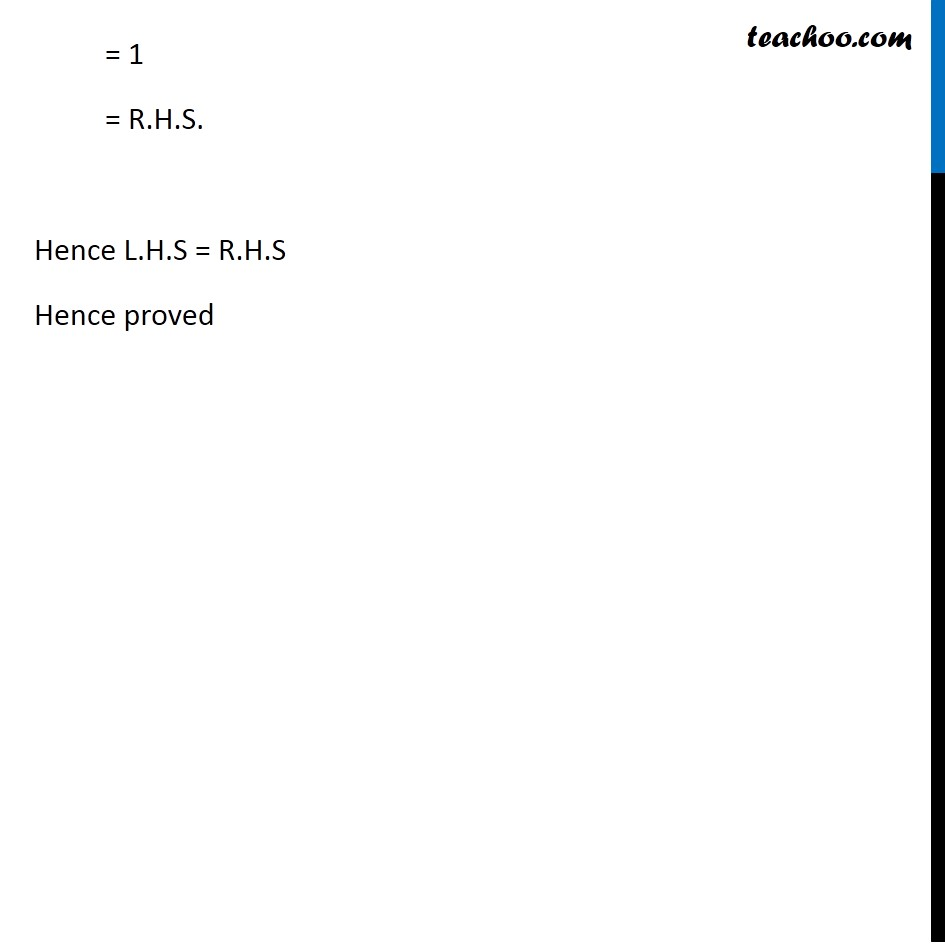1. Chapter 3 Class 11 Trigonometric Functions (Term 2)
2. Concept wise
3. (x + y) formula

Transcript

Ex 3.3, 22 Prove that cot 𝑥 cot 2𝑥 – cot 2𝑥 cot 3𝑥 – cot 3𝑥 cot 𝑥 = 1 Taking L.H.S. cot x cot 2x – cot 2x cot 3x – cot 3x cot x = cot x cot 2x – cot 3x (cot 2x + cot x) = cot x cot 2x – cot (2x + x) (cot 2x + cot x) = cot x cot 2x – ((cot 2x cot x − 1)/(cot x + cot 2x)) (cot 2x + cot x) = cot x cot 2x – (cot 2x cot x – 1) = cot x cot 2x – cot 2x cot x + 1 = 1 = R.H.S. Hence L.H.S = R.H.S Hence proved